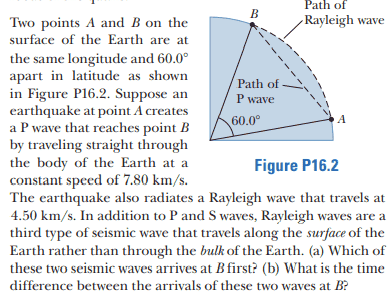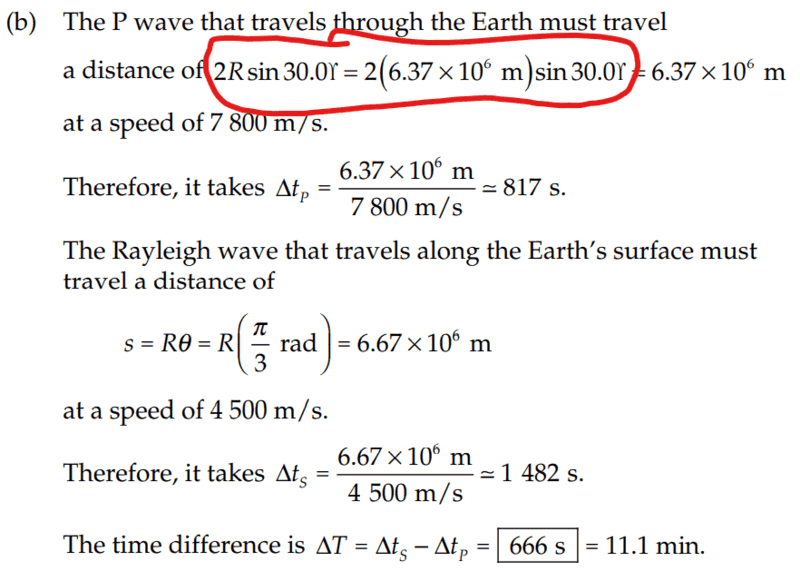# Finding time difference between two arriving wave fronts

Callumnc1
Homework Statement:
Relevant Equations:
S = Rθ
For part(b),The solution is,However, where did they get the formula shown in red from?

Many thanks!

#### Attachments

Homework Helper
Gold Member
##AB=2R\sin30^o.## Do you see why? Hint: Draw a perpendicular from the center to the base AB. It splits AB into two equal parts. What is the length of each part?

•Callumnc1
Callumnc1
##AB=2R\sin30^o.## Do you see why? Hint: Draw a perpendicular from the center to the base AB. It splits AB into two equal parts. What is the length of each part?
Thanks I see it now @kuruman ! Is the reason why they used radians instead of degrees in the arc length formula is because radians is a ratio of the length while degrees is not.

Many thanks!

Homework Helper
Gold Member
What you call the arc length formula is actually the definition of the angle as the ratio of the arc length to the radius. As such it has no dimensions.

•Callumnc1
Callumnc1
Thanks @kuruman ! But isn't degrees have no dimensions too?

Many thanks!

•